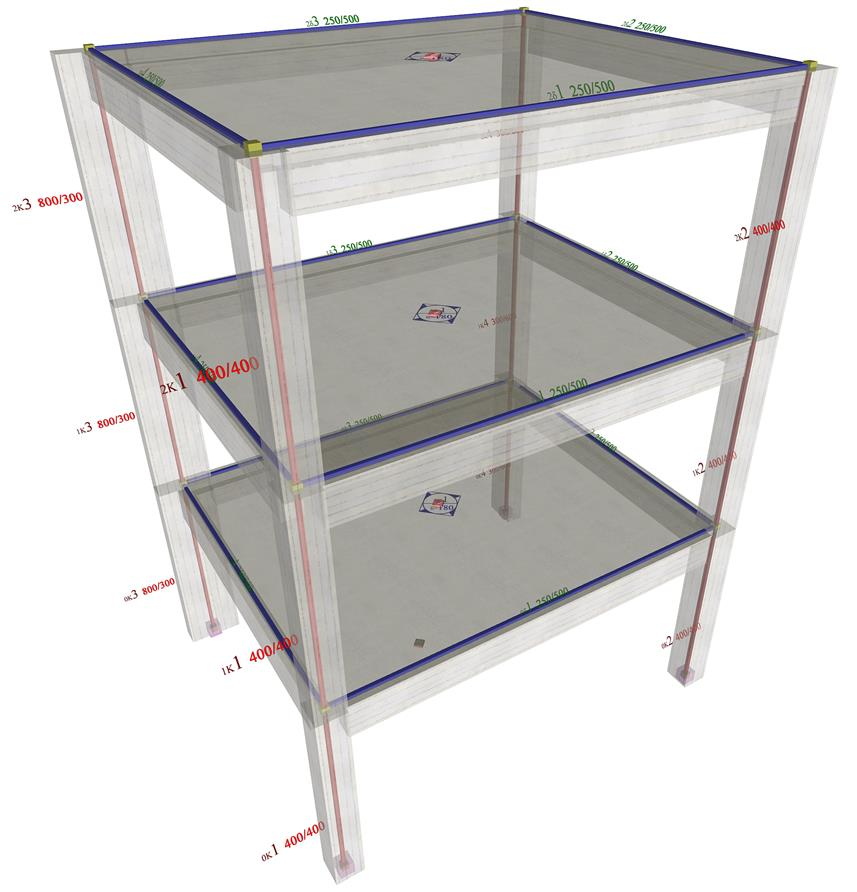« One-storey space frame with rectangular columns in parallel arrangement Exercises » Multistorey space frame of rectangular columns in parallel arrangement In this paragraph the analysis of the multistorey space frame illustrated in the figure, under horizontal seismic force, is performed using four methods: (i) analysis using manual calculations, assuming fixed-ended columns, (ii) analysis using the Excel file, assuming fixed-ended columns, (iii) analysis using the Excel file, assuming columns with k=6 and (iv) analysis using software, assuming actual beam and column torsional stiffnesses.Figure 5.4.6: The structure and the model of a three-storey space frame Figure 5.4.6: The structure and the model of a three-storey space frame The names, the loadings, the coordinates and the dimensions of slabs and columns are shown in figure of §5.4.1. The cross-section of beams is 250/500. The concrete class is C30/37 (Ε=32.80 GPa). Analysis using manual calculations, assuming fixed-ended columns (k=12) When a storey is typical throughout the building and all the columns are assumed to be fixed-ended then the typical storey is analyzed applying the theory for the one-storey structure described in the previous paragraphs of this chapter. . Analysis using the Excel file, assuming fixed-ended columns (k=12) The same spreadsheet, , is used since the same problem is concerned. The results are identical to those corresponding to the manual calculations. The structure,the torsional ellipse and the equivalent columns are drafted at the end of the spreadsheet. The results are identical to those of §5.4.5.2. Analysis using the Excel file, assuming columns with k=6 Changing the k factor of all columns from 12 to 6 in the Excel spreadsheet, makes no difference for the centre of stiffness which remains identical as for k=12. , The torsional radii and the stress resultants remain the same as those of the previous cases. Only the displacements change, whose relative values, of course, remain constant and equal to 12/6=2.0. The results are identical to those of §5.4.5.3. Analysis using software, assuming actual beam and column torsional stiffnesses This case cannot be solved by means of manual calculations. The use of software is required and in fact it goes back to the general case which is described in Appendix D, where, in general, the angles a of the principal systems of the storeys are non-zero. In Appendix D a relevant example is also presented for the verification of the algorithms of the general method. « One-storey space frame with rectangular columns in parallel arrangement Exercises »